MatLab Toolbox - LTE                                      Home : www.sharetechnote.com

Generating Time Domain Signal

In this posts, I will show you the process to convert the frequency domain OFDM Symbol into time doing data which is transmitted by the antenna. This posts would show you the all the process from the beginning to the end of Downlink process.

< SISO - Generating Time Domain Data >

% First you have to define properites of a eNodeB.

% NDLRB indicate System Bandwith in the unit of RBs.

% NDLRB 6 = 1.4 Mhz, NDLRB 15 = 3.0 Mhz, NDLRB 25 = 5.0 Mhz,

% NDLRB 50 = 10 Mhz, NDLRB 75 = 15 Mhz, NDLRB 100 = 20 Mhz

% CellRefP indicate number of downlink Antenna. CellRefP = 1 means 1 transmission antenna (SISO)

% NCellID indicate PCI (Physical Channel Identity) of the Cell

% NSubframe indicate the subframe number.

% In this example, most of these parameters are kept same for all subframe, but NSubframe value will be

% changed. so NSubframe will be set within the for loop.

enb.CyclicPrefix = 'Normal';

enb.PHICHDuration = 'Normal';

enb.Ng = 'Sixth';

enb.NDLRB = 6;

enb.CellRefP = 1;

enb.DuplexMode = 'FDD';

enb.NFrame = 0;

enb.NCellID = 0;

enb.CFI = 1;

% Now I will create an array variabel that will store all the subframe. It is initiailized with the empty array.

txFrameGrid = [];

% Now I will create a simple for loop and within the for loop I put the routine for creating all the signal and

% channel creation routing for each subframe. I just copied the whole routine from PDSCH creation example.

for subframeNo = 0:9   % start of for loop

% As I said, the enb paramters that does not change for each subframe is placed out side of the for loop

% I only put the chaning parameter (NSubframe) in this example within the forloop and associate it with

% for loop counter. Of course, which parameter you want to change for each subframe is up to you.

enb.NSubframe = subframeNo;

% Now populate all the information in DCI field as you like. Understanding details of DCI is also pretty

% huge topics. You would need separate page for DCI for the details.

dci.NDLRB = enb.NDLRB;

dci.DCIFormat = 'Format1A';

dci.AllocationType = 0;

dci.Allocation.RIV = 18;

dci.ModCoding = 10;

dci.HARQNo = 0;

dci.NewData = 0;

dci.TPCPUCCH = 0;

dci.DuplexMode = 'FDD';

dci.NTxAnts = 1;

% once you defined all the detailed fields of DCI, just pass it to lteDCI() function with eNB info as follows,

% then you will get the bit stream for the DCI.

[dciMessage,dciMessageBits] = lteDCI(enb,dci);

% for this step, you need to set a couple of additional parameters as shown below.

% C_RNTI will be XORed to CRC bits

% PDCCHFormat will determined Aggregation Level.

%          PDCCHFormat 0 indicate Aggregation Level 1

%          PDCCHFormat 1 indicate Aggregation Level 2

%          PDCCHFormat 2 indicate Aggregation Level 4

%          PDCCHFormat 3 indicate Aggregation Level 8

C_RNTI = 100;

pdcchConfig.RNTI = C_RNTI;

pdcchConfig.PDCCHFormat = 0;

% then pass dciMessageBits and pdcchConfig to lteDCIEncode, the you would get the encoded bitstream.

codedDciBits = lteDCIEncode(pdcchConfig, dciMessageBits);

% If you pass the enb into ltePDCCHInfo() function, it will give you the amount of resources that can be

% allocated for PDCCH allocation. This is not the amount of resource for only one DCI. It will give you the

% total/maximum amount of the resources that can be allocated for PDCCH.

pdcchDims = ltePDCCHInfo(enb);

% With ltePDCCHSpace, you can get the list of all the possible spaces that can carry PDCCH.

% In this example, the space were shown in the unit of bits.

pdcchBits = -1*ones(pdcchDims.MTot, 1);

% generate an array with the length that can accommodate all the possible PDCCH bits.

candidates = ltePDCCHSpace(enb, pdcchConfig, {'bits', '1based'});

% select one of the candidate bitSection and assign the codedDcitBits. You can select any candidate bit

%vsection, but in this example, I selected the first candidate section.

pdcchBits ( candidates(1, 1) : candidates(1, 2) ) = codedDciBits;

% if pass the encodedBits into ltePDCCH(), it will generate the modulated physical layer symbols.

pdcch_sym = ltePDCCH(enb, pdcchBits);

pdcch_sym_ind = ltePDCCHIndices(enb,{'1based','re'});

pdcch_sym_arrayIndex = 0:length(pdcch_sym)-1;

% Now we set various parameters defining PDSCH channel. (In real transmission, you would need to create

% a dci that is corresponding the configuration here. But in this example, I will go without defining DCI)

pdsch.NTxAnts = 1;

pdsch.NLayers = 1;

pdsch.TxScheme = 'Port0';

pdsch.Modulation = {'16QAM'};

pdsch.RV = 0;

pdsch.RNTI = C_RNTI;

% Now I have to create a vector carrying the number of PRB indexes that will be used to carry this PDSCH.

% for example, pdsch_prbs in following section would create a vector [0 1 2 3]

START_RB = 0;

N_RB = 4; %enb.NDLRB;

pdsch_prbs = (START_RB:(START_RB+N_RB-1)).';

% Now we have to generate a bit sequence which would exactly fit to the number of resource elements

% that are allocated for PDSCH for this specific subframe.

% To figure out exact Resource Element information, unlike in other channel processing, I would run

% ltePDSCHIndices() first. As you see in the following code, ltePDSCHIndices() returns the information that

%vwould give you the size of transport block size in the unit of bits.

[pdsch_sym_ind,pdschIndInfo] = ltePDSCHIndices(enb,pdsch,pdsch_prbs,{'1based','re'});

codedTrBlkSize = pdschIndInfo.G;

% now I would create a bit array that carries the user data. In this example, I generated randomly but

% in real situation, this would carry your user data (e.g, image, movie, files etc)

dlschTransportBlk = round(rand(1,codedTrBlkSize));

% now if you pass all the information to lteDLSCH), it will generate the encoded codeword data for the

% transport block you defined.

codeword = lteDLSCH(enb,pdsch,codedTrBlkSize,dlschTransportBlk);

% now if you pass the encoded data (codeword) with eNB and pdsch config to ltePDSCH(), you can

% generate physical layer symbols for the encoded data.

pdsch_sym = ltePDSCH(enb,pdsch,codeword);

pdsch_sym_arrayIndex = 0:length(pdsch_sym)-1;

% Following is to create an empty resource grid for one subframe.

resourceGrid = lteDLResourceGrid(enb);

% Following is to create symbols for Cell Specific Reference Signal and make a list of resource index for the

% reference signal.

rsAnt0 = lteCellRS(enb,0);

indAnt0 = lteCellRSIndices(enb,0);

resourceGrid(indAnt0) = rsAnt0;

% Following is to create symbols for PBCH and make a list of resource index for the signal (channel)

mib_bits = lteMIB(enb);

bch_cw = lteBCH(enb,mib_bits);

pbch_sym = ltePBCH(enb,bch_cw);

pbch_sym_arrayIndex = 0:length(pbch_sym)-1;

pbch_sym_ind = ltePBCHIndices(enb,{'1based','re'});

% Following is to create symbols for PSS and make a list of resource index for the signal

pss = ltePSS(enb);

pss_arrayIndex = 0:length(pss)-1;

pss_sym_ind = ltePSSIndices(enb,0,{'1based','re'});

% Following is to create symbols for SSS and make a list of resource index for the signal

sss = lteSSS(enb);

sss_arrayIndex = 0:length(sss)-1;

sss_sym_ind = lteSSSIndices(enb,0,{'1based','re'});

% Following is to create symbols for PCFICH and make a list of resource index for the signal

cfi_cw = lteCFI(enb);

pcfich_sym = ltePCFICH(enb,cfi_cw);

pcfich_sym_arrayIndex = 0:length(pcfich_sym)-1;

pcfich_sym_ind = ltePCFICHIndices(enb,{'1based','re'});

% Following is to create symbols for PHICH and make a list of resource index for the signal

PHICH_Group_Index = 0;

PHICH_Sequence_Index = 1;

HARQ_Indicator_Value = 0; % 0 = NACK, 1 = ACK

phich_sym = ltePHICH(enb,[PHICH_Group_Index,PHICH_Sequence_Index,HARQ_Indicator_Value]);

phich_sym_arrayIndex = 0:length(phich_sym)-1;

phich_sym_ind = ltePHICHIndices(enb,{'1based','re'});

% Following part is filling the resource grid with each of the signal..

pss_scale = 1.0;

sss_scale = 1.0;

phich_scale = 1.0;

pcfich_scale = 1.0;

pbch_scale = 1.0;

pdcch_scale = 1.0;

pdsch_scale = 1.0;

resourceGrid(pss_sym_ind) = pss_scale .* pss;

resourceGrid(sss_sym_ind) = sss_scale .* sss;

resourceGrid(pcfich_sym_ind) = pcfich_scale .* pcfich_sym;

resourceGrid(phich_sym_ind) = phich_scale .* phich_sym;

resourceGrid(pbch_sym_ind) = pbch_scale .* pbch_sym(1:length(pbch_sym_ind));

resourceGrid(pdcch_sym_ind) = pdcch_scale .* pdcch_sym;

resourceGrid(pdsch_sym_ind) = pdsch_scale .* pdsch_sym;

% Now We just completed the process of creating a subframe.

% Next step is to store this subframe to the variable we created for storing the whole radio frame.

txFrameGrid = [txFrameGrid resourceGrid];

end; % end of for loop

% Converting the whole radio frame into time domain data can be done by a single function lteOFDMModulate()

% as shown below.

% lteOFDMModulate() performs the following steps.

%    i) append zero pad to make the frequency domain data to be Nfft (Number of IFFT bins) for the specific BW

%    ii) perform IFFT

%    iii) add the CP (Cyclic Prefix)

[tx_waveform,tx_waveform_info] = lteOFDMModulate(enb,txFrameGrid);

% The time domain data is stored in tx_waveform. If you take one slot length of tx_waveform, the structure

% would be as follows. The number of samples shown here indicates the case for 20 Mhz BW. If the BW changes,

% the number of samples may vary. You can figure out the number of samples for a specific BW from

% tx_waveform_info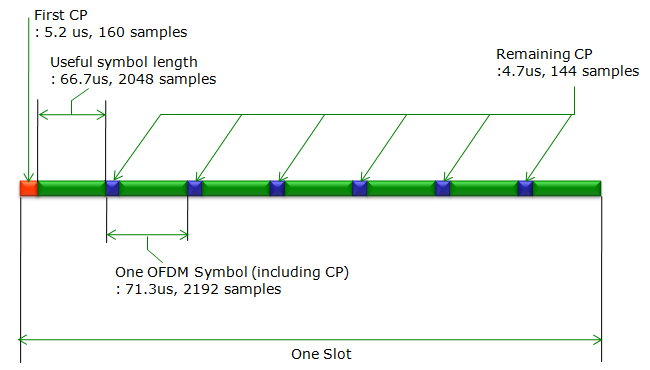< Plotting Time Domain Data for Each OFDM Symbol >

The data stored in tx_waveform in previous section carries the time domain data for a whole radio frame (10 ms) including the cyclic prefix. In this section, I will show you how to cut out a specific slot (there are 20 slots in a radio frame) and represents it in both frequency and time domain.

A couple of important thing that you have to consider is that the sampling rate varies with system bandwidth and the number of samples within an OFDM symbol is different between the first symbol and the rest of symbols within a slot.

The overall purpose of this section is to display the following structure into each separate OFDM symbols.% [160+2048;144+2048;144+2048;144+2048;144+2048;144+2048;144+2048]; represents the number of

% samples of Cyclic Prefix and OFDMA symbol data for each symbol with reference to 20 Mhz case show above

% (2048 IFFT bins, 30.702M sampling rate). For example, in case of 160 + 2048, 160 is the number of

% samples of the cyclic prefix of the first slot and 2048 is the number of samples of OFDMA symbol of the first

% slot.

% If System BW changes, the number of samples for data and cyclic prefix gets different. To figure out the

% number of samples for a specific BW from the 20 Mhz data, I create a variable SamplingScale

% SamplingScale is to scale the 20 Mhz case to the current BW that is being used.

SamplingScale = (double(tx_waveform_info.Nfft)/2048);

NoOfSampleList = SamplingScale * [160+2048;144+2048;144+2048;144+2048;144+2048;144+2048;144+2048];

% this is to convert the number in NoOfSampleList to represents the index number of each slot boundary.

SymbolSampleIndex = cumsum(NoOfSampleList);

% each elements of SymbolSampleIndex indicate the array index of the following positions.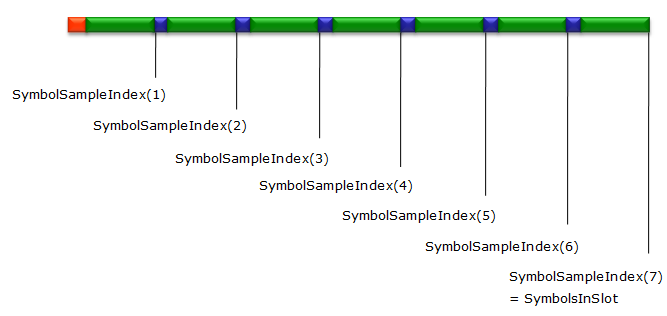% this is just to get the total number of data within a slot and store it in SymbolsInSlot

SymbolsInSlot = max(SymbolSampleIndex );

% You can specify the slot number for which you want to plot. since tx_waveform in this example carries 1 radio

% frame data, you can specifyvthe number 0~19 to this variable.

SlotNo = 2;

% tx_waveform contains all the samples within a radio frame in a single long one-dimensional array.

% In order to take out a chunk of a block of data corresponding to a specific OFDM symbol. We have to

% calculate the start and end of the sample index of the symbol. SymbolSampleIndexStart is the variable

% to store the index of the first sample in an OFDM symbol.

SymbolSampleIndexStart = 1;

% SymbolLength is the variable to store the number of samples contained in an OFDM symbol.

% Note that I used the function double() function to match the value type.

SymbolLength = double(tx_waveform_info.Nfft);

% Following for loop is to take out each OFDM symbol data from a specified slot and present it in plots.

% Each iteration will create two plots. The left plot would presents frequency domain plot for an OFDMA symbol

% the right plot would presents the time domain plot for an OFDMA symbol

for i = 1:7

% plotIndex stores the array of the index within tx_waveform corresponding to all the samples for a specified

% OFDM Symbol.

plotIndex = (SymbolSampleIndex(i)+(1-SymbolLength:0)) + (SlotNo * SymbolsInSlot) ;

% plotIndex for each 'i' value contains the indices for each symbol excluding Cyclic Prefix as shown below.% This is to take out all the samples for a specified (single) OFDM symbol and store the cut-out samples

% to the variable symbolData

symbolData=tx_waveform(plotIndex);

symbolPlotData = 0.0 * (1:length(tx_waveform));

symbolPlotData(1:length(symbolData)) = symbolData;

% Following is to convert the time domain data to frequency domain data.

freqData=fftshift(fft(symbolData));

% Following is to plot of frequency domain data on the left side graph.

subplot(7,5,5*(i-1)+1);

plot(abs(freqData)); xlim([1 length(symbolData)]);%ylim([0 0.2]);

set(gca,'xticklabel',[]); set(gca,'yticklabel',[]); set(gca,'xtick',[]); set(gca,'ytick',[]);

% Following is to plot of time domain I-data on the left side graph.

subplot(7,5,[5*(i-1)+2 5*(i-1)+3]);

plot(real(tx_waveform(plotIndex))); xlim([1 length(plotIndex)]);ylim([-0.25 0.25]);

set(gca,'xticklabel',[]); set(gca,'yticklabel',[]); set(gca,'xtick',[]); set(gca,'ytick',[]);

ylabel(strcat('S',num2str(i-1),'-I'));

% Following is to plot of time domain Q-data on the left side graph.

subplot(7,5,[5*(i-1)+4 5*(i-1)+5]);

plot(imag(tx_waveform(plotIndex))); xlim([1 length(plotIndex)]);ylim([-0.25 0.25]);

set(gca,'xticklabel',[]); set(gca,'yticklabel',[]); set(gca,'xtick',[]); set(gca,'ytick',[]);

ylabel(strcat('S',num2str(i-1),'-Q'));

% Following is to move the value of SymbolSampleIndexStart to the start sample index of next OFDM Symbol

SymbolSampleIndexStart = SymbolSampleIndex(i) + 1;

end;

Following example shows frequency and time domain I/Q data for the slot 0 (the first slot in 1st subframe) for System BW = 1.4 Mhz and PDSCH resource allocation of 4 RBs starting from RB 0. Examine each symbol plot (especially for frequency domain plot) and try to understand why you get such a plot.

 enb.NDLRB = 6; START_RB = 0; N_RB = 4; SlotNo = 0;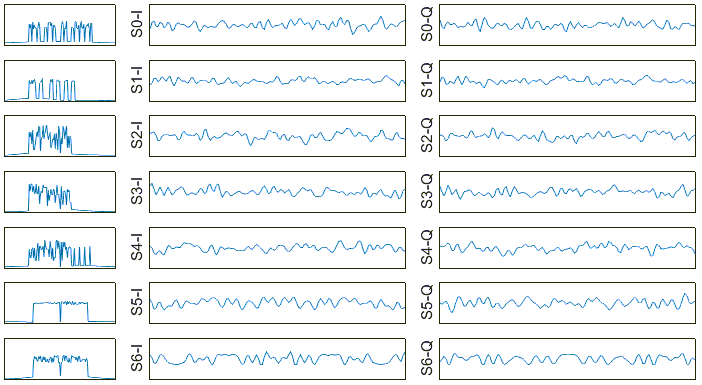Following example shows frequency and time domain I/Q data for the slot 1 (the second slot in 1st subframe) for System BW = 1.4 Mhz and PDSCH resource allocation of 4 RBs starting from RB 0. Examine each symbol plot (especially for frequency domain plot) and try to understand why you get such a plot. Do you see any difference between this example and previous example ? What is the difference ? why you have such a difference ?

 enb.NDLRB = 6; START_RB = 0; N_RB = 4; SlotNo = 1;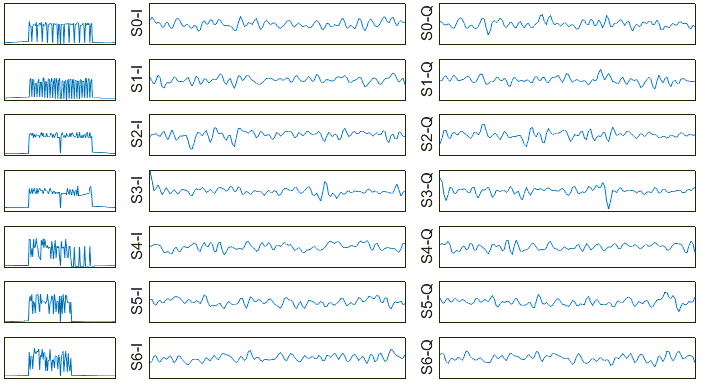Following example shows frequency and time domain I/Q data for the slot 2 (the first slot in 2nd subframe) for System BW = 1.4 Mhz and PDSCH resource allocation of 4 RBs starting from RB 0. Examine each symbol plot (especially for frequency domain plot) and try to understand why you get such a plot. Do you see any difference between this example and previous example ?

 enb.NDLRB = 6; START_RB = 0; N_RB = 4; SlotNo = 2;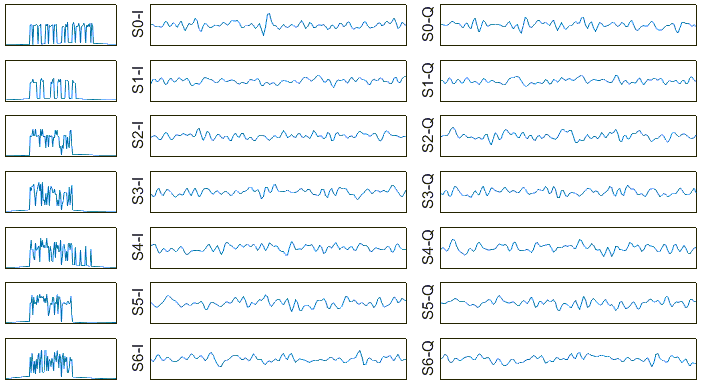Following example shows frequency and time domain I/Q data for the slot 0 (the first slot in 1st subframe) for System BW = 5 Mhz and PDSCH resource allocation of 4 RBs starting from RB 0. Examine each symbol plot (especially for frequency domain plot) and try to understand why you get such a plot.

 enb.NDLRB = 25; START_RB = 0; N_RB = 4; SlotNo = 0;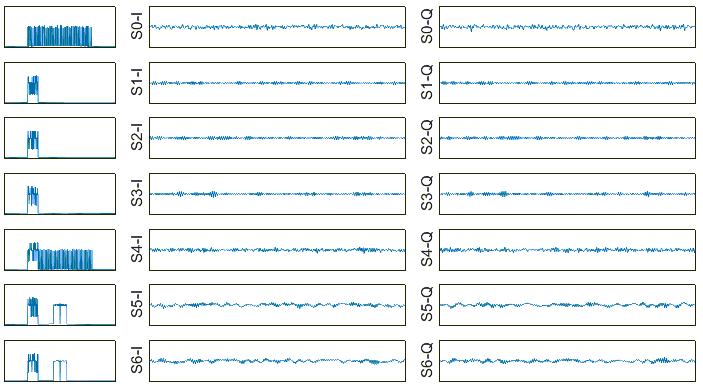Following example shows frequency and time domain I/Q data for the slot 1 (the second slot in 1st subframe) for System BW = 5 Mhz and PDSCH resource allocation of 4 RBs starting from RB 0. Examine each symbol plot (especially for frequency domain plot) and try to understand why you get such a plot. Do you see any difference between this example and previous example ? What is the difference ? why you have such a difference ?

 enb.NDLRB = 25; START_RB = 0; N_RB = 4; SlotNo = 1;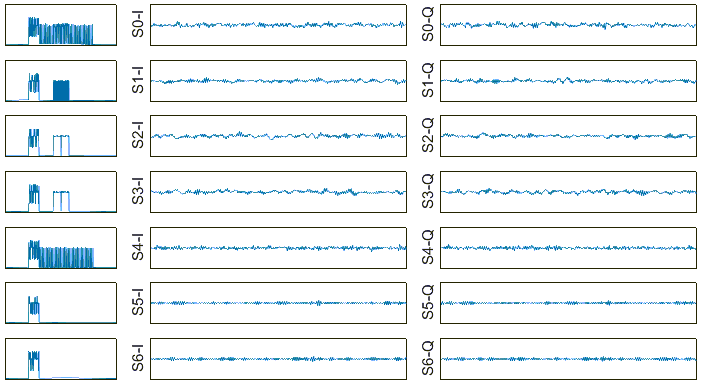Following example shows frequency and time domain I/Q data for the slot 2 (the first slot in 2nd subframe) for System BW = 5 Mhz and PDSCH resource allocation of 4 RBs starting from RB 0. Examine each symbol plot (especially for frequency domain plot) and try to understand why you get such a plot. Do you see any difference between this example and previous example ?

 enb.NDLRB = 25; START_RB = 0; N_RB = 4; SlotNo = 2;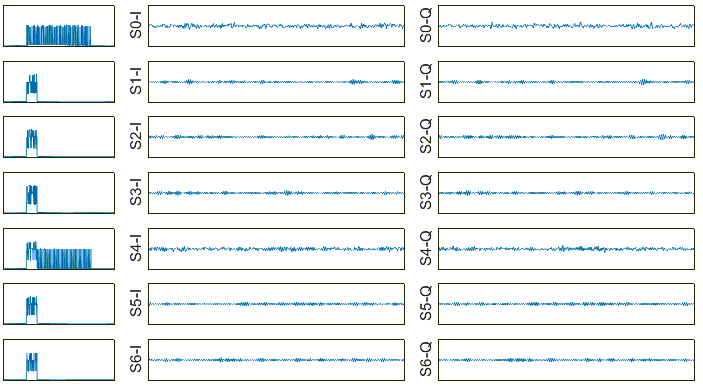< Plotting Resource Grid and Time Domain for whole Radio Frame >

This is a extension of the previous section. In previous section, I plotted the time domain and frequency domain for each OFDM symbol separately for one slot. In this section, I will repeat the previous process for all slots (20 slots) within a radio frame and plot them all together.

% [160+2048;144+2048;144+2048;144+2048;144+2048;144+2048;144+2048]; represents the number of

% samples of Cyclic Prefix and OFDMA symbol data for each symbol with reference to 20 Mhz case

% (2048 IFFT bins, 30.702M sampling rate). For example, in case of 160 + 2048, 160 is the number of

% samples of the cyclic prefix of the first slot and 2048 is the number of samples of OFDMA symbol of the first

% slot.

% SamplingScale is to scale the 20 Mhz case to the current BW that is being used.

SamplingScale = (double(tx_waveform_info.Nfft)/2048);

NoOfSampleList = SamplingScale * [160+2048;144+2048;144+2048;144+2048;144+2048;144+2048;144+2048];

% this is to convert the number in NoOfSampleList to represents the index number of each slot boundary.

SymbolSampleIndex = cumsum(NoOfSampleList);

% this is just to get the total number of data within a slot and store it in SymbolsInSlot

SymbolsInSlot = max(SymbolSampleIndex );

% Create three variables (empty arrays) to store the frequency domain, I/Q data

SpectrumList = [];

TimeDomainListI = [];

TimeDomainListQ = [];

% You can specify the slot number for which you want to plot.

% Since tx_waveform in this example carries 1 radio frame data, you can specify the number 0~19 to this

% variable.

for SlotNo = 0:19

% tx_waveform contains all the samples within a radio frame in a single long one-dimensional array.

% In order to take out a chunk of a block of data corresponding to a specific OFDM symbol. We have to

% calculate the start and end of the sample index of the symbol. SymbolSampleIndexStart is the variable

% to store the index of the first sample in an OFDM symbol.

SymbolSampleIndexStart = 1;

% SymbolLength is the variable to store the number of samples contained in an OFDM symbol.

% Note that I used the function double() function to match the value type.

SymbolLength = double(tx_waveform_info.Nfft);

% Following for loop is to take out each OFDM symbol data from a slot and do following procedure

%       i) convert it to frequency domain and add the data to SpectrumList[]

%       ii) take out I component and add the data to TimeDomainListI[]

%       iii) take out I component and add the data to TimeDomainListQ[]

for i = 1:7

% plotIndex stores the array of the index within tx_waveform corresponding to all the samples

% for a specified OFDM Symbol.

plotIndex = (SymbolSampleIndex(i)+(1-SymbolLength:0)) + (SlotNo * SymbolsInSlot) ;

% This is to take out all the samples for a specified (single) OFDM symbol and store the cut-out samples

% to the variable symbolData

symbolData=tx_waveform(plotIndex);

symbolPlotData = 0.0 * (1:length(tx_waveform));

symbolPlotData(1:length(symbolData)) = symbolData;

% Following is to convert the time domain data to frequency domain data.

freqData=fftshift(fft(symbolData));

% add (store) the frequency domain data to SpectrumList[]

SpectrumList = [SpectrumList ; abs(freqData)'];

% add (store) the time domain I data to TimeDomainListI[]

TimeDomainListI = [TimeDomainListI;real(tx_waveform(plotIndex))'];

% add (store) the time domain Q data  to TimeDomainListQ[]

TimeDomainListQ = [TimeDomainListQ;imag(tx_waveform(plotIndex))'];

% Following is to move the value of SymbolSampleIndexStart to the start sample index of next OFDM

% Symbol

SymbolSampleIndexStart = SymbolSampleIndex(i) + 1;

end;

end;

% Following is to plot the resource grid for the whole radio frame.

subplot(2,2,[1 2]);

surface(SpectrumList','FaceColor','flat','EdgeColor','none'); zlim([0 3.5]); xlim([1 140]); ylim([1 SymbolLength]); view([0,90]);

% Following is to plot time domain I data for the whole radio frame.

subplot(2,2,3);

waterfall(TimeDomainListI'); zlim([-0.25 0.25]); ylim([1 140]);

ylim([1 SymbolLength]); xlabel('Symbol index'); ylabel('Sample index');  view([-40,45]);

% Following is to plot time domain Q data for the whole radio frame.

subplot(2,2,4);

waterfall(TimeDomainListQ'); zlim([-0.25 0.25]); ylim([1 140]);

ylim([1 SymbolLength]); xlabel('Symbol index'); ylabel('Sample index');  view([-40,45]);

Following is the example for plotting frequency and I/Q data plot for the whole radion frame for BW = 1.4 MHz and and PDSCH resource allocation of 4 RBs starting from RB 0

 enb.NDLRB = 6; START_RB = 0; N_RB = 4;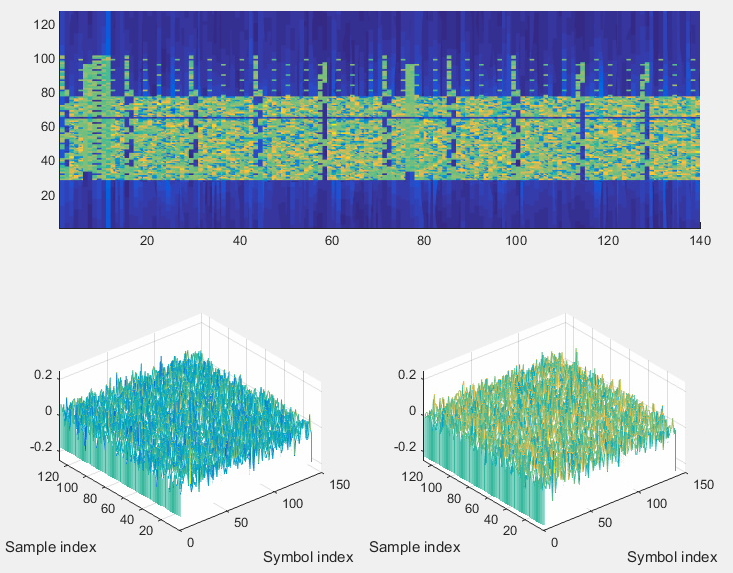Following is the example for plotting frequency and I/Q data plot for the whole radion frame for BW = 5 MHz and and PDSCH resource allocation of 4 RBs starting from RB 0

 enb.NDLRB = 25; START_RB = 0; N_RB = 4;< Plotting Frequency Spectrum  >

In this last section, I will show you how to display the radio frame time domain data stored in tx_waveform in spectrum analyzer. Method is very simple. Just pass the data into dsp.SpectrumAnalyzer(), but I added a short procedure to figure out the frequency span from enb parameter.

% following is to figure out RF bandwidth in Hz from enb parameter (enb.NDLRB)

if enb.NDLRB == 6

span = 1.4e6;

end;

if enb.NDLRB == 15

span = 3.0e6;

end;

if enb.NDLRB == 25

span = 5.0e6;

end;

if enb.NDLRB == 50

span = 10.0e6;

end;

if enb.NDLRB == 75

span = 15.0e6;

end;

if enb.NDLRB == 100

span = 20.0e6;

end;

% Following is to create a spectrum analyzer instances with proper configuration for displaying the tx_waveform

% Green part is something you have to pay attention and may want to change as you want.

hSpecAnalTx = dsp.SpectrumAnalyzer('SampleRate', tx_waveform_info.SamplingRate, ...

'SpectrumType', 'Power density', 'PowerUnits', 'dBm', ...

'RBWSource', 'Property','RBW', 1.5e3, ...

'FrequencySpan', 'Span and center frequency', ...

'Span', span, 'CenterFrequency', 0, ...

'Window', 'Hamming', 'SpectralAverages', 20, ...

'YLimits', [-100 -40], 'YLabel', 'PSD', ...

'Title', 'LTE Downlink Transmission Spectrum', ...

'ShowLegend', false);

% Following is to pass the data into spectrum analyzer that was created above.

step(hSpecAnalTx, tx_waveform);

 enb.NDLRB = 6; enb.CellRefP = 1; enb.NCellID = 0; START_RB = 0; N_RB = 4; tx_waveform_info =     SamplingRate: 1920000             Nfft: 128        Windowing: 4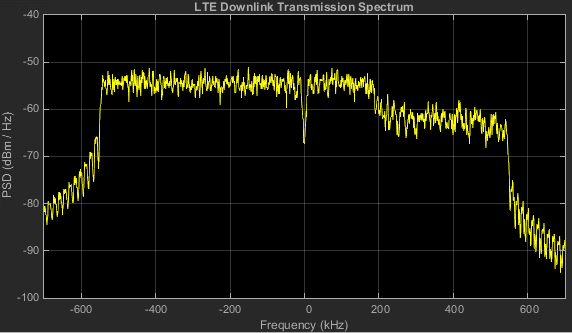Disclaimer ! :

This page is only to show you the overall logics and visualization for various LTE physical layer channels. I haven't investigated much about verifying about the accuracy.

If you think the code is not so efficient, it is 100% my fault. I haven't made any effort for effiecient code. I just tried to create code as simple as possible for the readers. As you know, easy-to-read code is not always efficient for a specific chipset.

If you find any mistake in terms of accuracy, it is also very highly likely be my fault. Not the problem of Matlab tool box itself.

Any comment and corrections if you find any mistake will be welcome and appreciated.